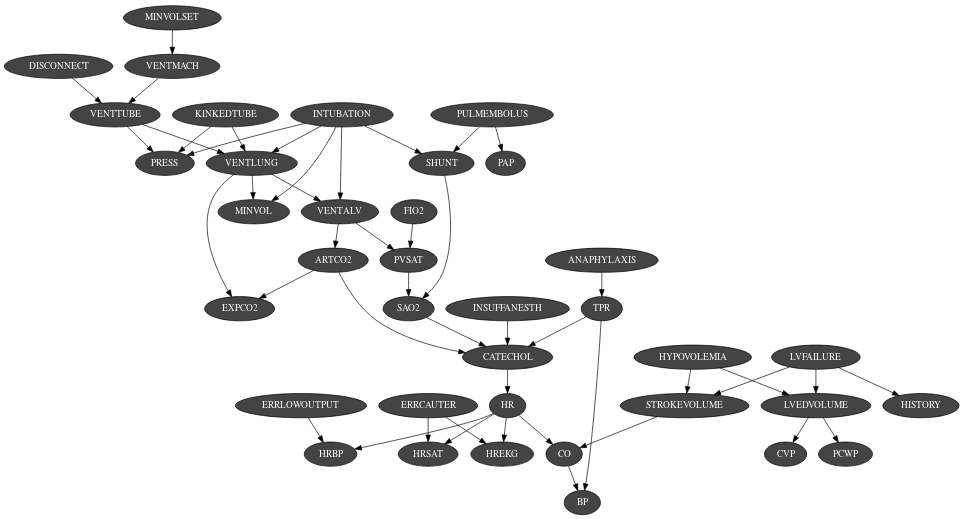# Bayesian Network¶The Bayesian Network is the main graphical model of pyAgrum. A Bayesian network is a directed probabilistic graphical model based on a DAG. It represents a joint distribution over a set of random variables. In pyAgrum, the variables are (for now) only discrete.

A Bayesian network uses a directed acyclic graph (DAG) to represent conditional indepencies in the joint distribution. These conditional indepencies allow to factorize the joint distribution, thereby allowing to compactly represent very large ones. Moreover, inference algorithms can also use this graph to speed up the computations. Finally, the Bayesian networks can be learnt from data.

Tutorial

Reference